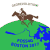# Power operator at the Band Arithmetic

72
3
a week agoNew Contributor

Hello! Maybe anybody knows how to use the power operator to create a formula to calculate an index based on the image bands. For example, I try to use formula: 0.5847*((B1**(-0.1294))*(B2**0.3389)*(B3**(-0.3118))).

3 RepliesOccasional Contributor II

That should work, if you make the assignments

B1 = Raster('rasterFile\Band_1')
B2 = Raster('rasterFile\Band_2')
B3 = Raster('rasterFile\Band_3')
index = 0.5847*((B1**(-0.1294))*(B2**0.3389)*(B3**(-0.3118)))

— AndyNew Contributor

Thank you for the solution. Can you explain where to create it, please? I mean what tool I should use if I work at the ArcGIS Pro 2.9.3.Occasional Contributor II

Simplest is the Python window, or if you want to save it for future reference you could create a Jupyter notebook; both are available in the ribbon Analysis. If you plan to use it repeatedly, though, I’d create a script in a toolbox, which would allow you to easily load different files as arguments: